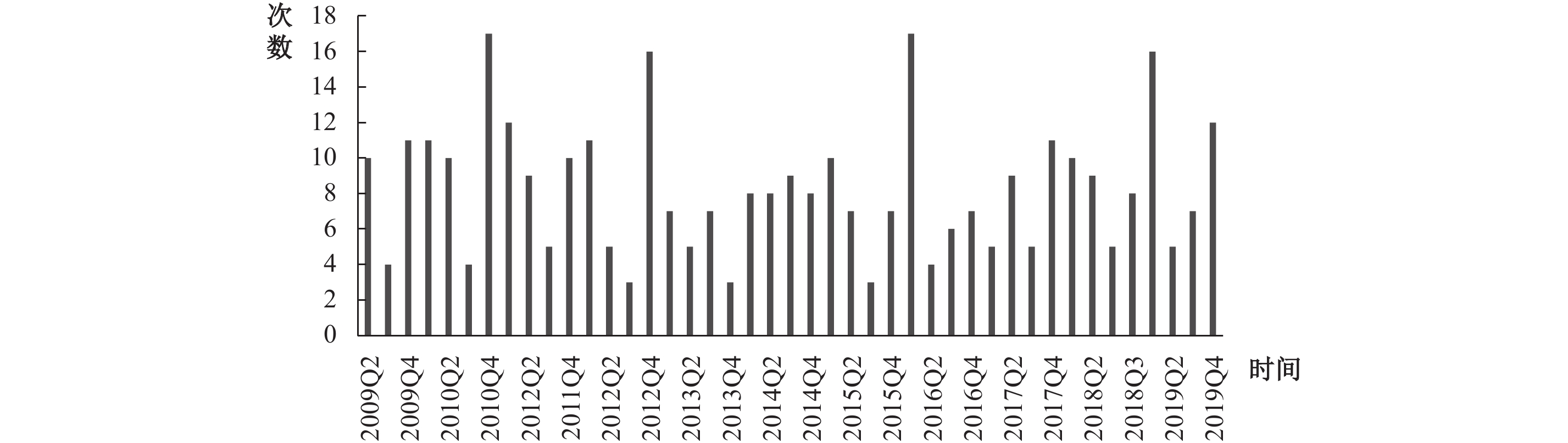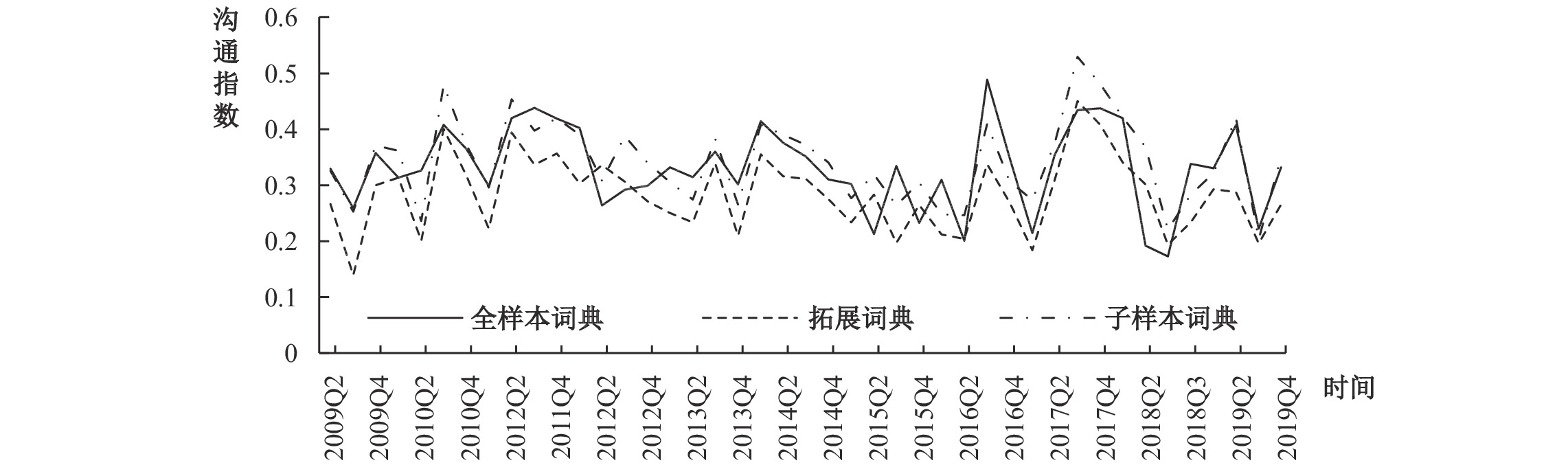﻿ 宏观审慎监管口头沟通与系统性风险《财经研究》
2021第47卷第7期

1. 湖南大学 金融与统计学院，湖南 长沙 410006;
2. 厦门大学 经济学院，福建 厦门 361005

Verbal Communication in Macro-prudential Supervision and Systemic Risk
Zhao Jing1, Xu Haiping2
1. School of Finance and Statistics，Hunan University，Changsha 410006，China;
2. School of Economics，Xiamen University，Xiamen 361005，China
Summary: The financial crisis in 2008 made countries recognize the great harm of financial systemic risk. After the crisis, all countries gradually realize the importance of macro-prudential supervision and adopt a variety of macro-prudential supervision tools to restrain systemic risk. As the prevention of financial systemic risk requires high timeliness and foresight, good communication plays a vital role in effective macro-prudential supervision. Therefore, countries should also improve the transparency of relevant macro-prudential policies in addition to using macro-prudential supervision tools. Countries have been using macro-prudential supervision verbal communication to guide the expectations of market entities, and scholars have been beginning to study its effect. However, there is a lack of research on whether verbal communication can effectively reduce the financial systemic risk in China. It is of great practical significance for China to improve the macro-prudential supervision framework and prevent financial systemic risk to measure the macro-prudential supervision verbal communication index reasonably and analyze its role in preventing financial systemic risk. This paper discusses the above issues. The result shows that the macro-prudential supervision authority will conduct verbal communication on changes in financial systemic risk. And the changes of incoming links and outgoing links in the sectors of bank, security, insurance and trusts have an effect on verbal communication. Macro-prudential supervision verbal communication helps to reduce financial systemic risk with a lag of about half a year. And it mainly affects bank incoming links and insurance outgoing links. Moreover, based on the supervised learning method, we train sub-sample dictionaries to obtain prone phrases and their probability distributions, use text classifiers to automatically classify new communication texts, and finally construct the index of macro-prudential supervision verbal communication on new samples. The result shows that the index results obtained by different samples are relatively consistent, indicating that the quantitative method of macro-prudential supervision verbal communication in this paper is relatively robust, reproducible and malleable. The expansion of this paper is mainly reflected in two aspects: First, considering the importance of foresight and timeliness in the prevention of financial systemic risk, this paper uses the text analysis method and the supervised learning method to construct the macro-prudential supervision verbal communication index from the perspective of verbal communication. It is more objective and reproducible. Second, this paper discusses the relationship between macro-prudential supervision verbal communication and financial systemic risk, which makes up for the lack of existing studies that mainly analyze the role of macro-prudential supervision tools and the impact of macro-prudential supervision verbal communication on financial markets.
Key words: macro-prudential supervision    verbal communication    dictionary analysis    supervised learning method    systemic risk

2008年国际金融危机使各国普遍认识到系统性金融风险的巨大危害。危机后，各国逐渐意识到宏观审慎监管的重要性。宏观审慎监管考虑了整个金融体系的稳定和金融机构间的相互影响，弥补了微观监管仅关注个体风险的不足。一方面，众多国家和国际组织纷纷设立专司宏观审慎监管的部门，以监控并防范系统性风险，如美国2010年设立了金融稳定监管委员会（Financial Stability Oversight CouncilFSOC）、欧盟2011年成立了欧洲系统性风险委员会（European Systemic Risk BoardESRB）、英国2013年设立了审慎监管局（Prudential Regulation AuthorityPRA）等；另一方面，众多国家和国际组织从时间和截面两个维度制定了多种宏观审慎监管工具（梁琪等，2015）。根据IMF（2011）对全球49个国家采用的宏观审慎工具的调查，其可以分为三类：第一类为信贷类，该类工具重点是为了约束借款人的贷款数量，包括贷款价值比、信贷总量或信贷增长、债务收入比、外汇贷款上限等；第二类为资本类，该类工具主要为了提高银行等金融机构的资本金以提升其抵御损失的能力，包括逆周期资本监管、杠杆率、动态拨备等；第三类为流动类，该类工具重点关注金融机构的流动性，包括货币错配、期限错配、净稳定资金比率、流动性覆盖率、准备金等（Lim等，2011；苗文龙和闫娟娟，2020）。然而，每个国家采用的具体的宏观审慎工具会因其金融与经济发展水平、监管效率等而有所不同（Lim等，2011），其中，逆周期资本要求、动态拨备等逆周期调整工具是多数国家常用的工具。此外，新兴市场国家更加关注系统流动性风险（systemic liquidity risk），经常使用流动类监管工具以及信贷总量或信贷增速限制等信贷工具，而发达国家更倾向于使用贷款价值比、债务收入比等信贷类工具。已有跨国研究发现，宏观审慎工具有助于缓解金融系统性风险的顺周期性问题（Lim等，2011；Claessens 等，2013；IMF，2015），可以有效约束信贷增长（IMF，2015；樊明太和叶思晖，2020），以及降低金融机构和市场的共同风险暴露（Lim等，2011），从而有效发挥降低系统性风险的作用。基于银行微观数据的研究也表明，宏观审慎工具有助于降低银行信贷增速（Claessens等，2013；梁琪等，2015）和杠杆率（Claessens等，2013；梁琪等，2015），从而可以降低系统性风险。也有部分研究发现，宏观审慎工具的作用效果会受一国的监督效率、宏观经济政策等的影响，不同类型工具的作用效果在不同国家会存在差异（Lim等，2011；樊明太和叶思晖，2020）。

(一) 自定义词典的生成

1. 文本收集。为了构建宏观审慎监管口头沟通指数，本文需要搜集人行、银保监会领导人在公开场合所发表的有关宏观审慎监管的内容。参考冀志斌和宋清华（2012）的研究，我们在百度新闻上搜集报道，以样本期内的人行行长和副行长、银保监会主席和副主席的姓名加上“影子银行”“业务复杂性”“关联”“脆弱性”“系统重要性机构”“金融稳定”“表外业务”“MPA”“宏观审慎”“系统性风险”“逆周期”“顺周期”“泡沫”等关键词，按时间顺序重点记录相关性高的口头沟通事件的时间、发言人、网址、沟通内容。为了提高基础词典的有效性，我们在搜索到相关的新闻报道后会到人行以及银保监会的官方网站找到相对应的领导讲话、演讲、采访等沟通事件原文，以避免语料中包含过多的媒体转述语言。同时，由于市场对相关领导人不定期发表在金融经济报刊上的文章的反应类似于口头沟通，且相比于《金融稳定报告》，这些文章篇幅较短，专业性不强，受众更广，因此本文同时记录了领导人在人行官方期刊《紫光阁》《中国金融》等期刊上发表的相关文章，使搜集的口头沟通事件更全面。为了保证实证结果的及时性和准确性，沟通时间以对应沟通事件原文所述时间为准；部分沟通事件若找不到相关原文，则以第一篇新闻报道的时间为准；而且为了避免重复测度，只选取沟通的原文或者第一次新闻报道。图 1 宏观审慎监管口头沟通事件频次图

2. 语料处理。本文主要聚焦于宏观审慎监管相关的口头沟通，但人行同时兼顾多种职能，其在进行宏观审慎监管口头沟通时往往不只针对系统性风险或宏观审慎监管发表看法，也经常包括其他沟通内容，如改进民生和社会发展等。为了防止其他内容的干扰，进一步提高准确度，本文首先将每次宏观审慎监管口头沟通事件中与经济无关的沟通内容剔除，再进行后续分析。

(二) 生成计算词典

$p_n^i = \dfrac{{f_n^i}}{{{f_n}}}$
。其中，
${f_n}$

$f_n^i$

 长度统计量 均值 标准差 中位数 众数 3.699 3.028 2 2 长度分布 短语长度 短语个数（占比） 短语长度 短语个数（占比） M $\in$ [2,4] 1702（81.36%） M $\in$ [10,19] 94（4.49%） M $\in$ [5,9] 284（13.58%） M $\in$ [20,35] 12（0.57%）

(三) 构建宏观审慎监管口头沟通指数

 $\frac{{\sum\nolimits_{n = 1}^N {p_{n,s}^i \times {f_{n,s}}} }}{{\sum\nolimits_{n = 1}^N {{f_{n,s}}} }}$ (1)

$p_{n,s}^i$

${f_{n,s}}$

$p_t^i = \dfrac{{\sum\nolimits_{{k_t}} {\left( {p_s^i} \right)kt} }}{{{k_t}}}$
。其中，
${k_t}$

$p_t^i$
t季度倾向为i类别的概率。

${I}_{t}$
。该指数的定义为t时期悲观的概率
${p}_{t}^{{\text{悲观}}}$

${p}_{t}^{{\text{乐观}}}$

${I}_{t}={p}_{t}^{{\text{悲观}}}-{p}_{t}^{{\text{乐观}}}$
${I}_{t}$
$\in$
[−1，1]。其中，
${I_t}$图 2 金融机构关联度指数与滞后2期宏观审慎监管口头沟通指数趋势图

(一) 金融机构关联度指数的构建

1. 估计金融机构的在险价值。首先，设立分位数回归方程如下：

 ${X_{j,t}} = {\alpha _{^j}} + {\gamma _{^j}}{M_{t - 1}} + {\varepsilon _{j,t}}$ (2)

${X_t} = 100 \times \ln({P_t}/{P_{t - 1}})$
Pt为金融机构的周收盘价。

 $Va{R_{j,t,q}} = {\hat \alpha _j} + {\hat \gamma _j}{M_{t - 1}}$ (3)

$Pr({X_{j,t}} \leqslant Va{R_{j,t,q}}) = q$

2. 构建金融机构间的关联度指数。本文将基于分位数回归和LASSO方法来构建金融机构间的相互联系网络。具体方程如下：

 ${X_{j,t}} = {\alpha _{j{{\left| W\! j\right.}}}} + \beta {_{{j{{\left| W \!j\right.}}}}^{L}{}^{ T}}{W_{j,t}} + {\varepsilon _{j,t}}$ (4)
 $CoVaR_{j{{\left| {\tilde W j,t,q} \right.}}}^L = {{\hat \alpha }_{j{{\left| {\tilde W j} \right.}}}} + \hat \beta {_{{j{{\left| {\tilde W j} \right.}}}}^{L}{}^{ T}}{{\tilde W}_{j,t}}$ (5)

${W_{j,t}}$

${M_{t - 1}}$
，金融机构特征变量
$B{C_{j,t - 1}}$
，其他金融机构的对数收益率
${X_{ - j,t}}{\rm{ = }}\left\{ {{X_{1,t}},{X_{2,t}}, \cdots ,{X_{k,t}}} \right\}$
，即除了金融机构j以外的所有其他金融机构的对数收益率，k代表金融机构的总数目。将所有其他金融机构的对数收益率纳入考虑，从而测度出金融机构间的风险传染效应。

${W_{j,t}}$

 $\min \frac{1}{T}\sum\limits_{t = 1}^T {{\rho _q}} \left( {{X_{j,t}} - {\alpha _{{j|{W j}}}} - \beta {{_{{j|{W j}}}^L}^{ T}}{W_{j,t}}} \right){\rm{ + }}{\lambda _j}\frac{{\sqrt {q(1 - q)} }}{T}\sum\limits_{k = 1}^K {{{\hat \sigma }_k}\left| {\beta _{{j|{W j,k}}}^L} \right|}$ (6)

${\lambda _j}$

${W_t} = \left( {{W_{k,t}}} \right)_{k = 1}^K$
，相应的方差为
$\hat \sigma _{kt}^2 = \frac{1}{T}\sum\nolimits_{t = 1}^T {{{\left( {{W_{k,t}}} \right)}^2}}$
；损失函数
${\rho _q}\left( u \right) = u\left( {q - I\left( {u < 0} \right)} \right)$

$I\left( {u < 0} \right)$

$\beta _{^{j|{W_j}}}^L{\rm{ = }}{\left\{ {\beta _{^{j| - j}}^L,\beta _{^{j|M}}^L\beta _{^{j|{B_j}}}^L} \right\}^T}$
，可以使用滚动窗口来估计不同窗口下的参数。相应的系数估计值为
$\hat \beta _{^{j|{{\tilde W}_j}}}^L{\rm{ = }}{\left\{ {\hat \beta _{^{j| - j}}^L,\hat \beta _{^{j|M}}^L\hat \beta _{^{j|{B_j}}}^L} \right\}^T}$
，回归系数
$\hat \beta _{^{j| - j}}^L$

$\hat \beta _{^{j|i}}^s$

$\hat \beta _{^{j| - j}}^s$

$T{C_s} = TC_s^{INL} = TC_s^{OUTL} = \sum\nolimits_{i = 1}^k {\sum\nolimits_{j = 1}^k {\left| {\hat \beta _{j|i}^s} \right|} }$

(二) 口头沟通与金融机构关联度指数的变化趋势

(三) 口头沟通与金融机构关联度的联动关系分析

 ${I}_{t}={\phi }_{o}+{ \sum \limits _{i=1}^{m}{\phi }_{i}{I}_{t-i}+}{ \sum \limits _{i=1}^{m}{\eta }_{i}{Y}_{t-i}+}{\varepsilon }_{2t}$ (7)
 ${Y}_{t}={\theta}_{o}+{ \sum \limits _{i=1}^{m}{\theta }_{i}{Y}_{t-i}+}{ \sum \limits _{i=1}^{m}{\delta }_{i}{I}_{t-i}+}{\varepsilon }_{3t}$ (8)

$GroupC_{g,s}^{IN} =$
$\sum\nolimits_{i = 1}^k {\sum\nolimits_{j \in g} {\left| {\hat \beta _{j|i}^s} \right|} }$
，各部门的传染指数为
$GroupC_{g,s}^{OUT} = \sum\nolimits_{i \in g} {\sum\nolimits_{j = 1}^k {\left| {\hat \beta _{j|i}^s} \right|} }$
，其中，g=1，2，3，4，分别代表银行、证券、保险和信托部门。然后，我们使用宏观审慎监管口头沟通指数和各部门的风险关联度月度数据来分析两者的联动关系。

2. 格兰杰因果关系检验。由表2的检验结果可知，“bankin不是I的格兰杰原因”的假设无法在10%的显著性水平下被拒绝，“I不是bankin的格兰杰原因”的假设在5%的显著性水平下被拒绝，表明宏观审慎监管口头沟通对银行业被传染指数的变化有较好的解释力；同时，前者检验的P值为13.7%，说明银行业被传染指数的变化对宏观审慎监管口头沟通具有一定的影响。“bankout不是I的格兰杰原因”的假设在5%的显著性水平下被拒绝，“I不是bankout的格兰杰原因”的假设无法被拒绝，即bankoutI之间存在单向格兰杰因果关系，表明银行业传染指数的变化对宏观审慎监管口头沟通有较好的解释力。“insurin不是I的格兰杰原因”的假设在1%的显著性水平下被拒绝，“I不是insurin的格兰杰原因”的假设无法被拒绝，即insurinI之间存在单向格兰杰因果关系，表明保险业被传染指数的变化对宏观审慎监管口头沟通有较好的解释力。“insurout不是I的格兰杰原因”检验的P值为11.8%，“I不是insurout的格兰杰原因”的假设被拒绝，即insurout与口头沟通I之间在一定程度上存在双向格兰杰因果关系，表明保险业传染指数变化与宏观审慎监管口头沟通会相互影响。“secin不是I的格兰杰原因”的假设在5%的显著性水平下被拒绝，“I不是secin的格兰杰原因”的假设无法被拒绝，即secinI之间存在单向格兰杰因果关系，表明证券业被传染指数的变化对宏观审慎监管口头沟通有较好的解释力。证券业传染指数也具有类似的结果，secoutI之间存在单向格兰杰因果关系，说明证券业传染指数的变化对宏观审慎监管口头沟通有较好的解释力。信托业结果与证券业类似，trustintrustout同样与口头沟通I之间存在单向格兰杰因果关系，说明信托业被传染指数和传染指数的变化对宏观审慎监管口头沟通都有较好的解释力。

 原假设 统计量 P 值 结果 原假设 统计量 P 值 结果 bankin 不是I 的格兰杰原因 5.520 0.137 接受 secin 不是I 的格兰杰原因 9.522 0.023 拒绝 I 不是bankin的格兰杰原因 8.556 0.036 拒绝 I不是secin 的格兰杰原因 1.655 0.647 接受 bankout 不是I的格兰杰原因 10.220 0.017 拒绝 secout 不是I 的格兰杰原因 6.963 0.073 拒绝 I 不是bankout的格兰杰原因 1.821 0.61 接受 I不是secout的格兰杰原因 2.947 0.4 接受 insurin不是I 的格兰杰原因 11.890 0.008 拒绝 trustin不是I 的格兰杰原因 9.794 0.02 拒绝 I不是insurin 的格兰杰原因 1.384 0.709 接受 I 不是trustin的格兰杰原因 0.617 0.893 接受 insurout 不是I 的格兰杰原因 5.870 0.118 接受 trustout 不是I的格兰杰原因 4.355 0.037 拒绝 I不是insurout 的格兰杰原因 9.064 0.028 拒绝 I 不是trustout 的格兰杰原因 4.322 0.229 接受

(一) 比较不同样本量信息对测度结果的影响

(二) 拓展词典

$p_{n,s}^i = \dfrac{{\sum\nolimits_{n = 1}^N {p_{n,s}^i \times {f_{n,s}}} }}{{\sum\nolimits_{n = 1}^N {{f_{n,s}}} }}$
，然后比较乐观、中性、悲观三个类别的概率。若乐观的概率最大，则将该句子自动归类于乐观沟通；其他类别的态度划分以此类推。图 3 不同词典下宏观审慎监管口头沟通指数趋势图

① 2021年4月15日，中国人民银行微信公众号发文《打好防范化解重大金融风险攻坚战 切实维护金融安全》，对近年来我国在防范化解重大金融风险方面取得的成效进行了总结。资料来源：https://mp.weixin.qq.com/s/LqO_rhVNwj-wund3gmvENQ

② 资料来源于中国人民银行宏观审慎管理部分：http://www.pbc.gov.cn/huobizhengceersi/214481/index.html

③ 限于篇幅，文中未列示金融机构关联度指数与当期、滞后1期、滞后3期宏观审慎监管口头沟通指数趋势图（备索）。